Project

# Embedded PID Temperature Control, Part 5: Adjusting Gains

February 29, 2016 by Robert Keim

## We will explore how P, I, and D gain influences system performance, and in the process we will find a gain configuration that works well for our temperature controller.

We will explore how P, I, and D gain influences system performance, and in the process, we will find a gain configuration that works well for our temperature controller.

### Previous Articles in This Series

Before we get started, here is the PID control system diagram presented previously: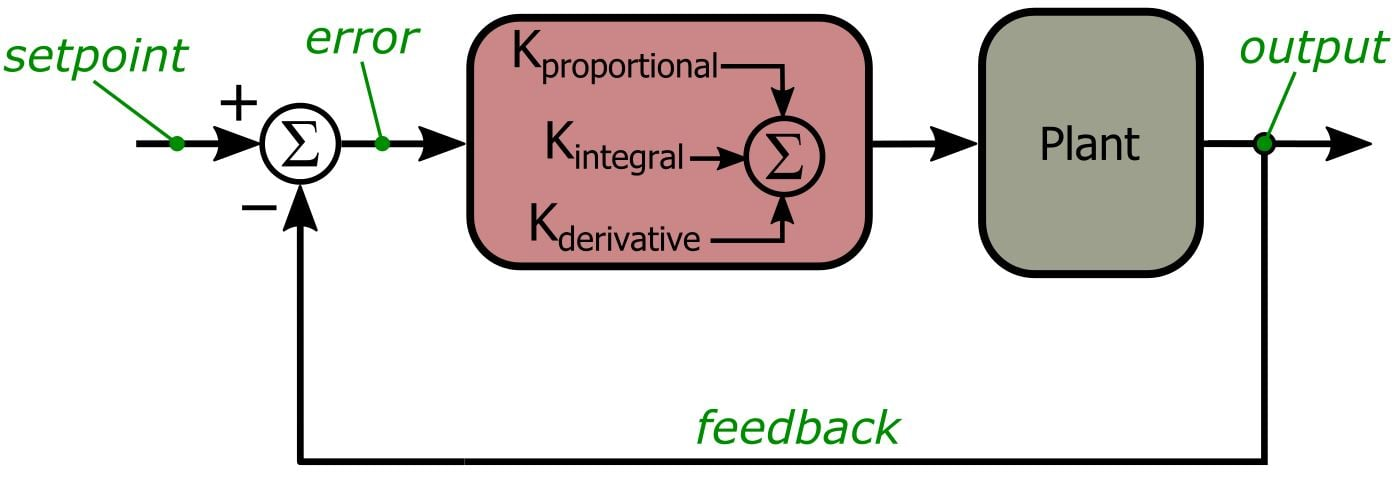And here are PID-related portions of the schematic: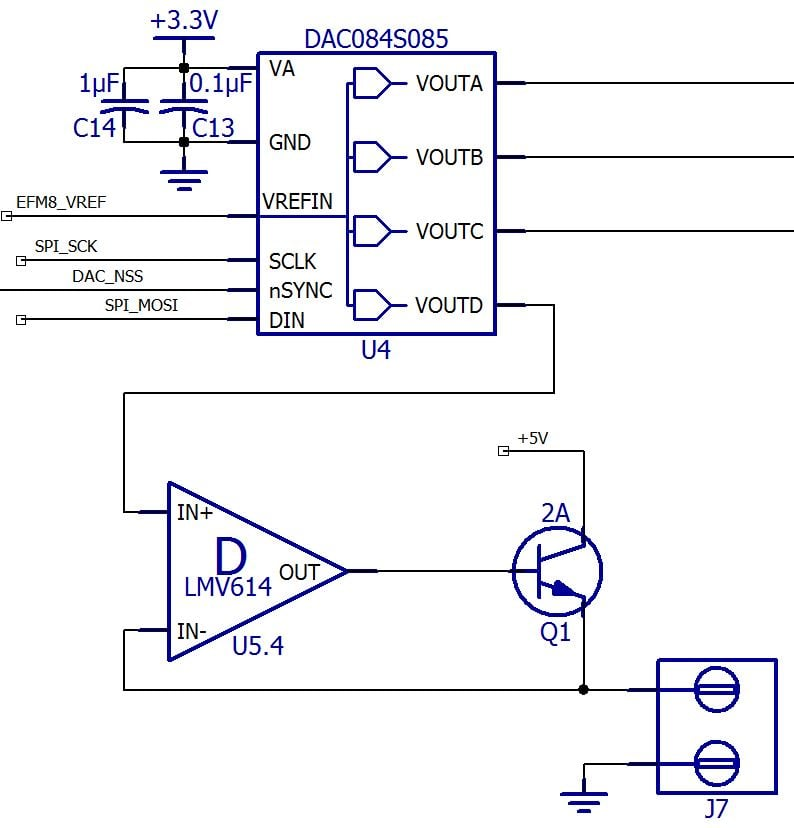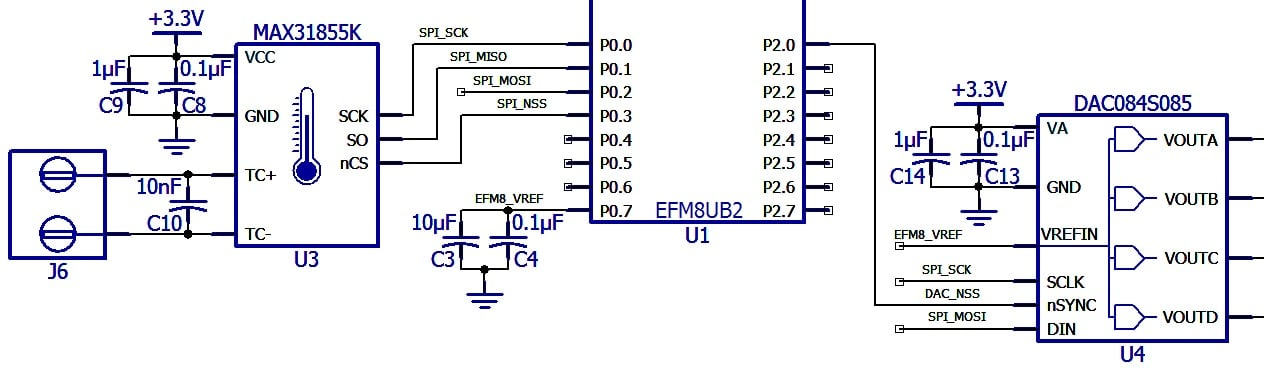Our goal in this article is to acquire a solid conceptual understanding of how proportional, integral, and derivative gain affects the performance of a PID control system. This will be a lot easier if we have a convenient way to change the gain values. Thus, we need to add some new functionality to the GUI: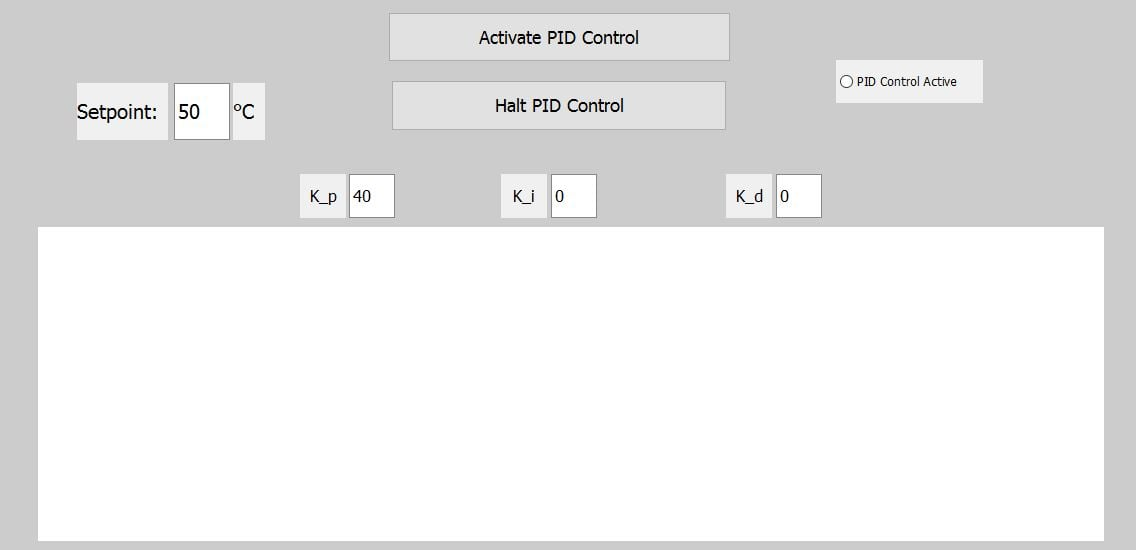As you can see, we now have text-entry boxes for P, I, and D gain. These values are sent to the EFM8 in the same way as the setpoint, i.e., via a USB command transmitted at the beginning of each control run (a “control run” starts when you click “Activate PID Control” and ends when you click “Halt PID Control”; the plot showing the measured temperatures and the setpoint line is cleared at the beginning of a new control run). The gain values are limited to integers in the range 0 to 255. Let’s take a quick look at two portions of the Scilab script that are relevant to this new functionality. Here we convert the digits in the text-entry boxes into variables that can be sent to the EFM8 as plain binary numbers (rather than ASCII characters):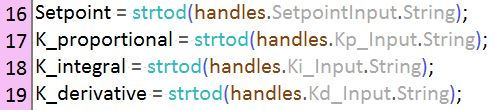And this is how we send the gain values to the EFM8. Previously we had only two transmissions here—an “S” command for sending the setpoint and a “C” command for initiating the control run. Now we have a third, namely, a “K” command for sending all three gain values.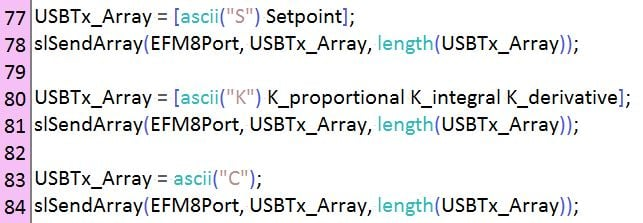PID_Temperature_Control_GUI_v2.zip

Of course, this new GUI feature will accomplish nothing if the EFM8 firmware doesn’t know what to do with a “K” command, so let’s briefly look at the firmware changes. First, we need to convert K_proportional, K_integral, and K_derivative from local variables defined in the main() function to global variables that will be available to both main() and VCPXpress_API_CALLBACK(). We do this by defining the variables outside of any function, and then we also declare them as “extern” in the Project_DefsVarsFuncs.h file. Now we can add some code for handling a “K” command: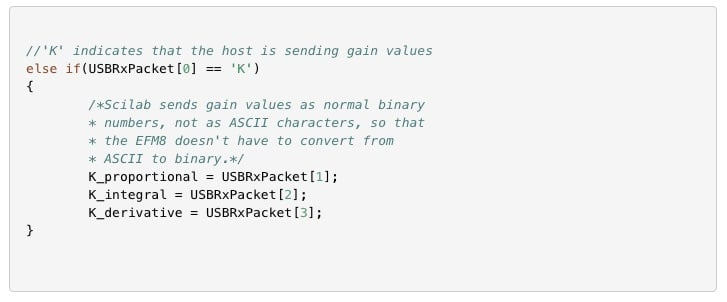Here is a link to download all the source and project files for the firmware used in this stage of the project.

PIDTemperatureControl_Part5.zip

To turn your PID code into something that actually controls a variable, you need to find a reasonable value for the P gain. This is not as straightforward as one might think, because in the context of a low-level, application-specific PID system (such as our temperature controller), the gain values are actually translating numerical information. What I mean is this: Our system has two separate numerical domains—temperature (in degrees Celsius) and digital counts (which in turn correspond to a DAC voltage, which in turn corresponds to a heater-drive current, which in turn corresponds to the amount of heat generated by the resistor). These two domains speak different languages; what we need to do is set the proportional gain such that it will properly translate from one numerical domain to the other.

You can do a decent job of this just by thinking about how exactly the system operates: We read a temperature value from the MAX31855 and calculate the error. This error then determines the amount of heat generated by the resistor. We get maximum heat at a DAC value of 200 (the system supports DAC values up to 255, but I chose 200 as the upper limit). We want maximum heat when the error is large, i.e., when the measured temperature is far from the setpoint. At some point, though, as the measured temperature approaches the setpoint, the heat output should start to decrease. This is where you just kind of pick a value that makes sense, then after a few control runs you can adjust it as necessary. Let’s say we want the heater to stay at max until the measured temperature is within 5°C of the setpoint: 200/5 = 40. So we start with a proportional gain of 40.

Remember that the PID control output is the DAC value, and to calculate the PID output we multiply the proportional gain by the “error,” which means the setpoint temperature minus the measured temperature. Thus, as long as the error is greater than or equal to 5°C, the heater is maxed out. As the measured temperature approaches the setpoint, the heater-drive current decreases in proportion to the error—at 4°C the DAC value is 160, at 3°C the DAC value is 120, and so forth. This is your starting point, and if necessary you can adjust the proportional gain based on the actual performance of the system. Once you are happy with the proportional gain, you can move on to integral and derivative gain.

### The P-Only System

Do you really need integral and derivative gain? Well, that depends on your operational requirements (and the characteristics of your system). Let’s look at a control run for a P-only system with KP = 40.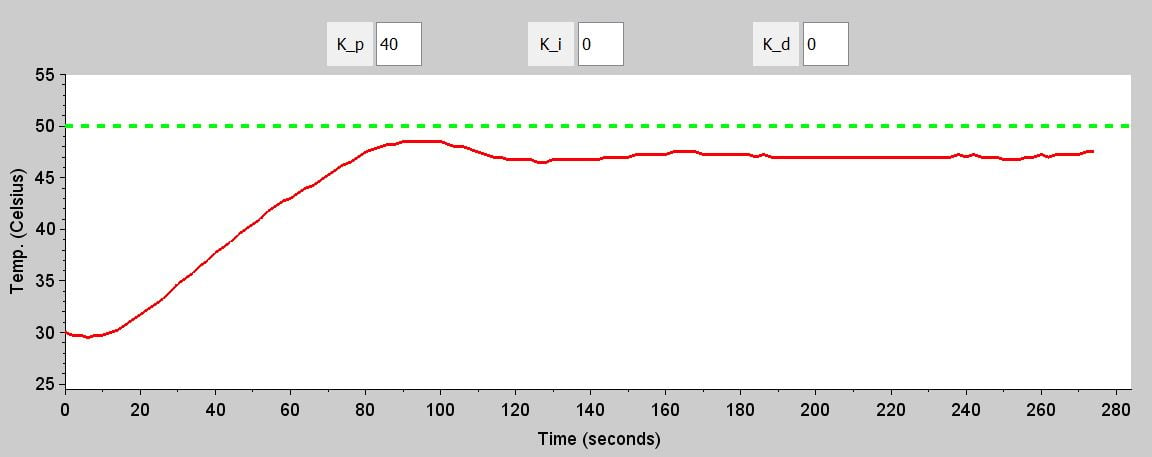As expected, the system suffers from significant steady-state inaccuracy. When the error is small, the P gain is not enough to counteract the resistor’s natural tendency to cool off toward the ambient temperature. Can we fix this problem simply by increasing the proportional gain? Well, here are four P-only control runs with KP = 70, 100, 150, and 200.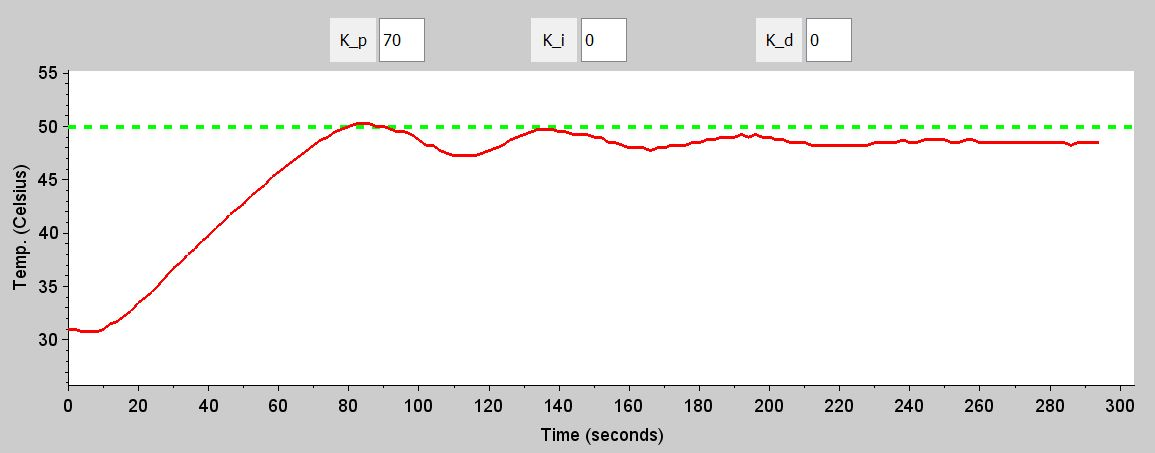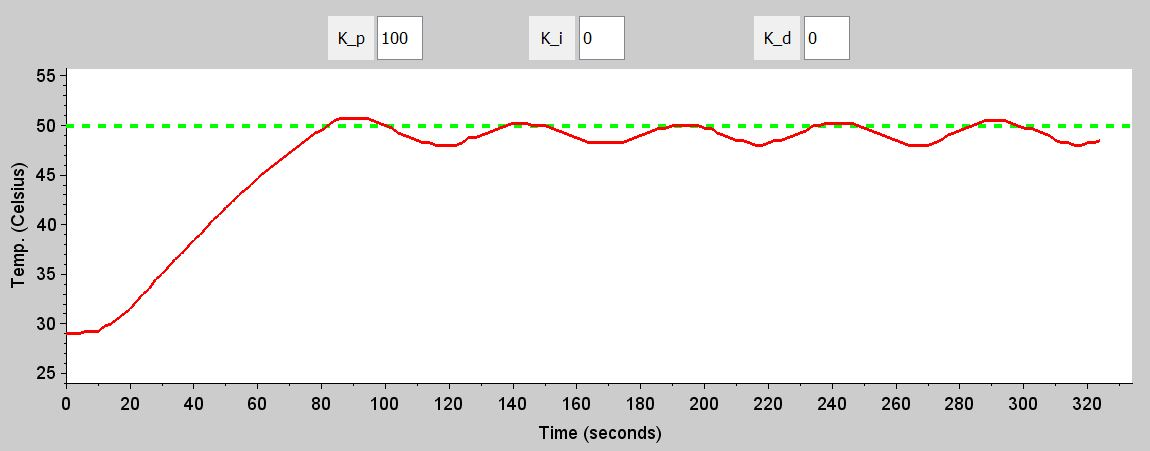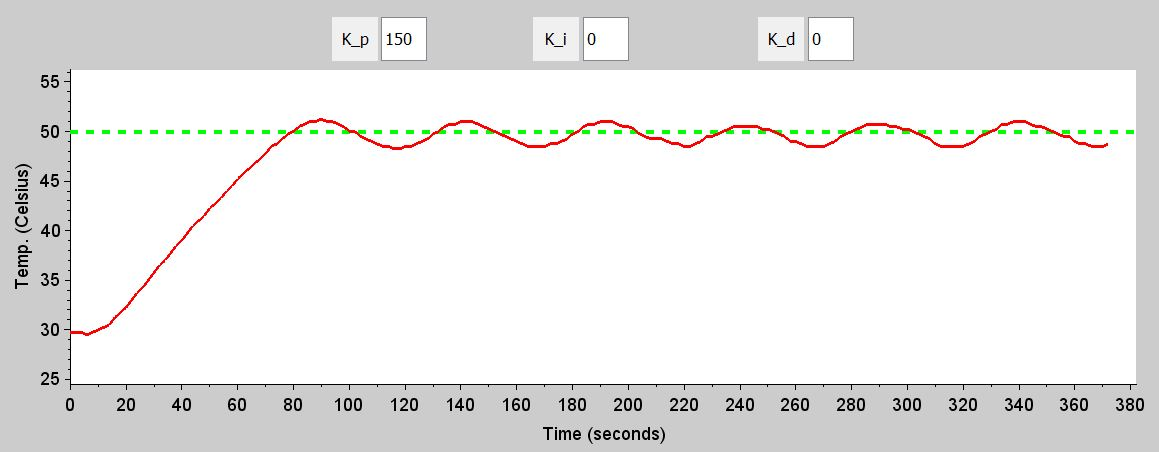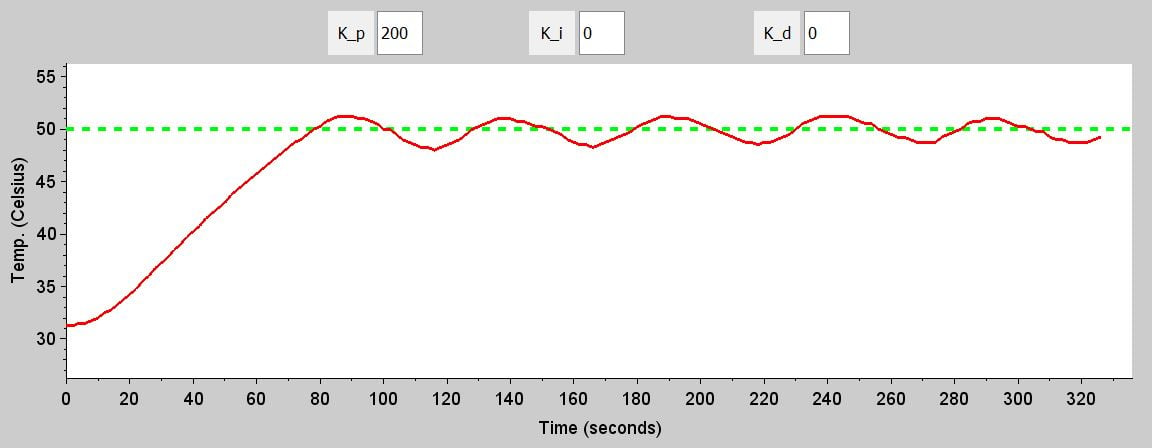Ponder these plots for a minute, and I think you will start to understand the weakness of a P-only system. If the gain is too low, you have a major steady-state error. As the gain gets higher, you simply exchange steady-state error for oscillation. By the time we get to KP = 200, the output is almost centered around the setpoint—in other words, the average steady-state error is very small—but we have sustained oscillations of fairly significant amplitude. If you are satisfied with this performance, I suppose you could stop here. But I’m not satisfied.

### Bring In the Integral

The integral term allows small errors to gradually accumulate and thus exert more influence on the PID output than they would in a P-only system. You have to be careful, though, because integral errors can accumulate quickly, and with too much integral gain the system starts to act like a pendulum—error builds up below the setpoint and drives the temperature too high, then error builds up above the setpoint and drives the temperature too low, then error builds up below the setpoint and drives the error too high, and so forth.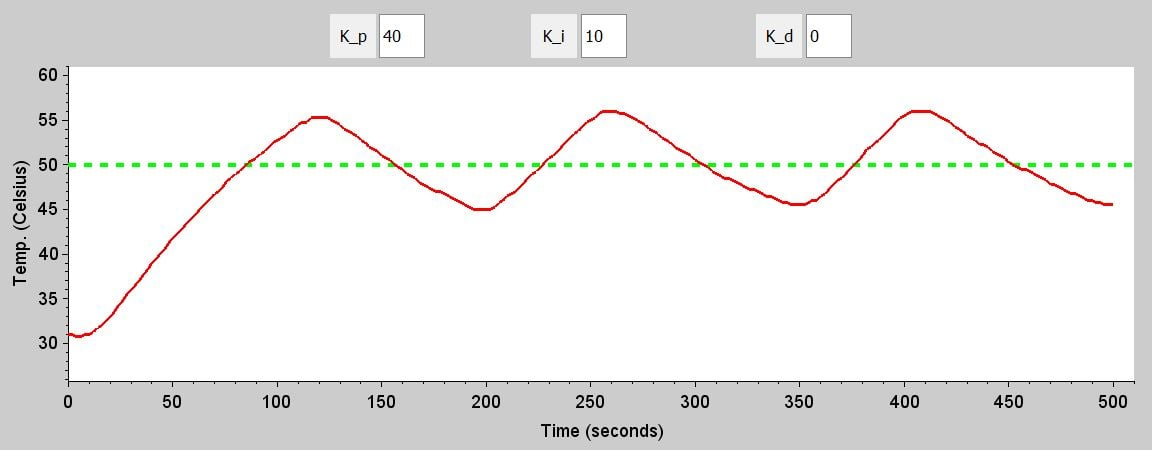Obviously, this value is too high because we have major pendulum behavior. (We know that the oscillation is caused by the integral gain, not the proportional gain, because the P-only system with KP = 40 did not oscillate.) Let’s try KI = 5.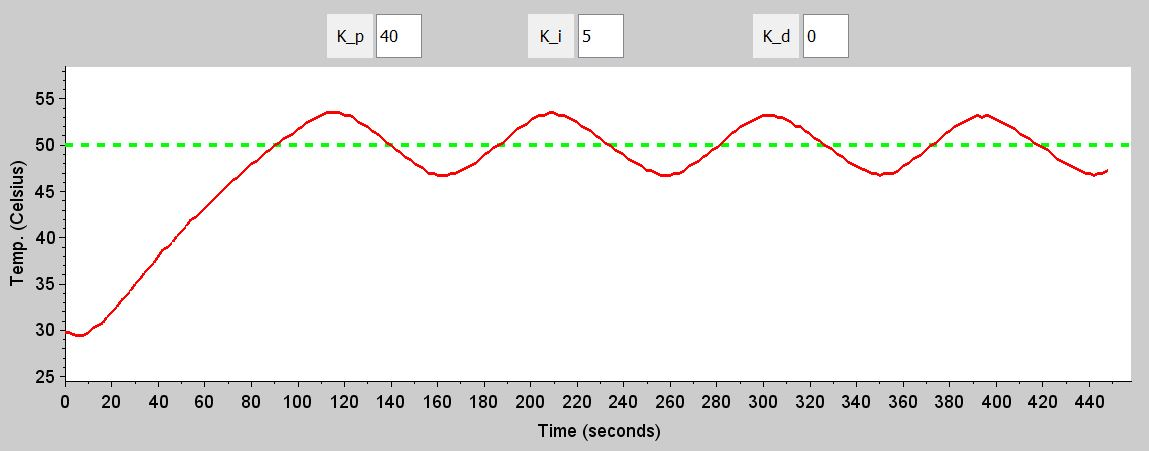This is still too much integral gain, but we’re getting closer—with KI = 10 the oscillations were about 11°C peak-to-peak, and with KI = 5 they are only about 6°C peak-to-peak. Here are the plots for control runs with KI = 3 and KI = 2.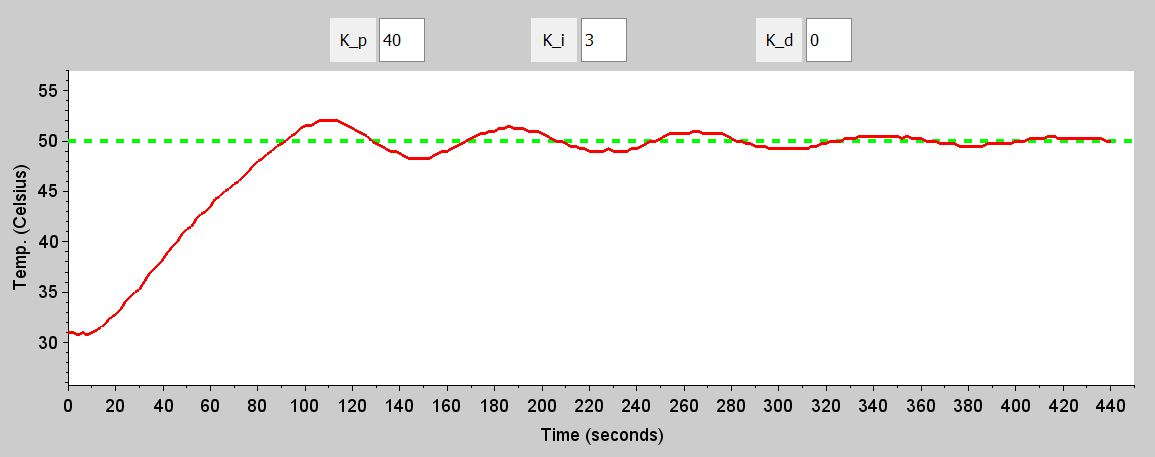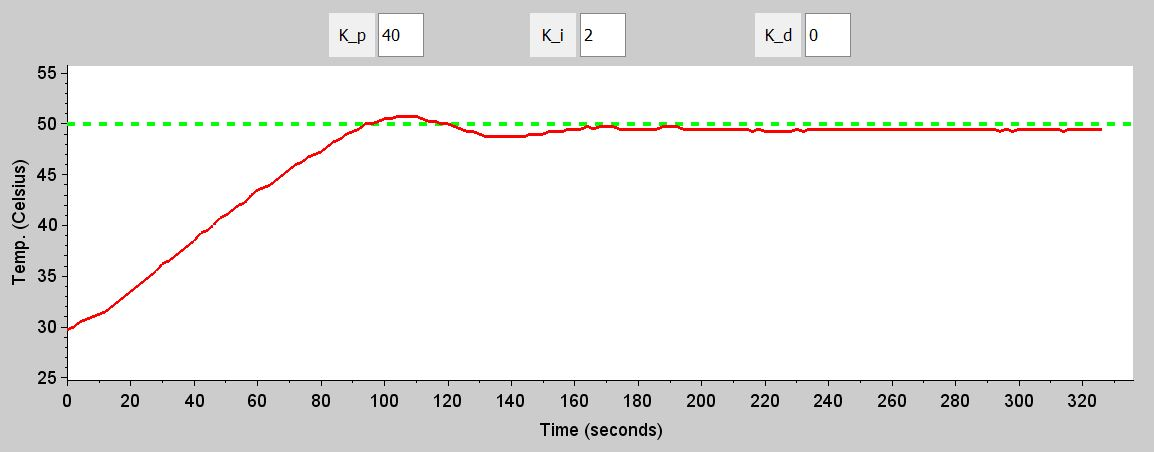Both of these are good. The KI = 3 run has significant oscillation but the amplitude is clearly decreasing, so we can assume that the measured temperature will eventually find the setpoint and stay there. The KI = 2 run doesn’t have oscillation, but there is not quite enough integral gain to overcome the steady-state-error tendency of the P-only system. At this point, I like the KP = 40/KI = 3 system, but it would be nice if the output converged more quickly. For this we need derivative gain.

### Finish with the Derivative

Many PID systems are actually implemented without derivative gain. As we saw in the previous section, PI controllers can be quite effective. Also, the derivative is susceptible to noise that causes the short-term rate of change to be inconsistent with the overall behavior of the output. But in general, a PID controller will not attain its full potential without some derivative gain. The derivative term makes the system more responsive because it modifies the PID output based on the future behavior of the system. Think about it this way: As the measured temperature approaches the setpoint, the error is getting smaller. Thus, the change in error (calculated as the current error minus the previous error) is negative. This change in error is then multiplied by the (positive) derivative gain value and added to the PID output. The result of the multiplication is negative, so it decreases the PID output—the derivative term reduces the PID output based on the expectation that the output is approaching the setpoint, and this leads to less overshoot. The reverse happens when the measured temperature is decreasing toward the setpoint: the derivative term increases the PID output as it approaches the setpoint and thus reduces undershoot.

Here are control runs for KD = 1, 2, and 3: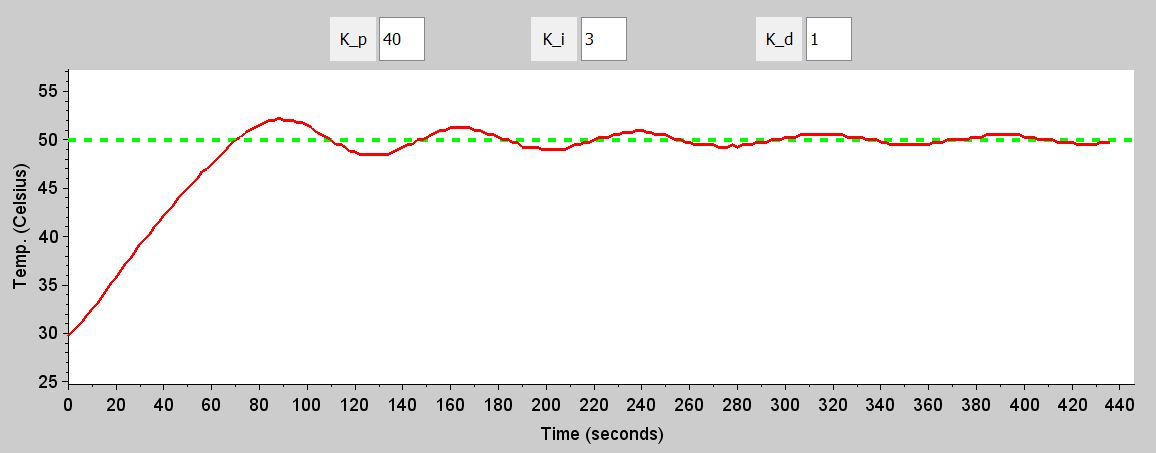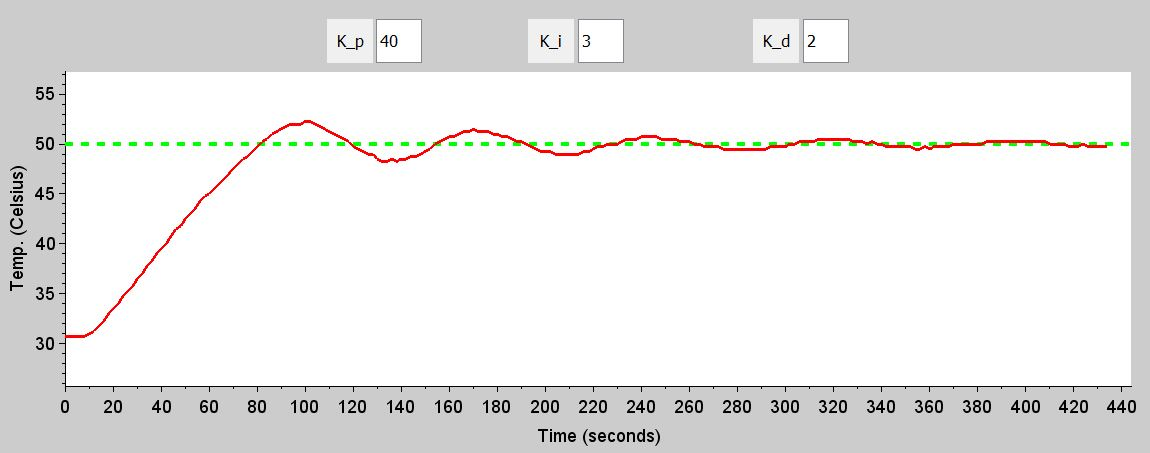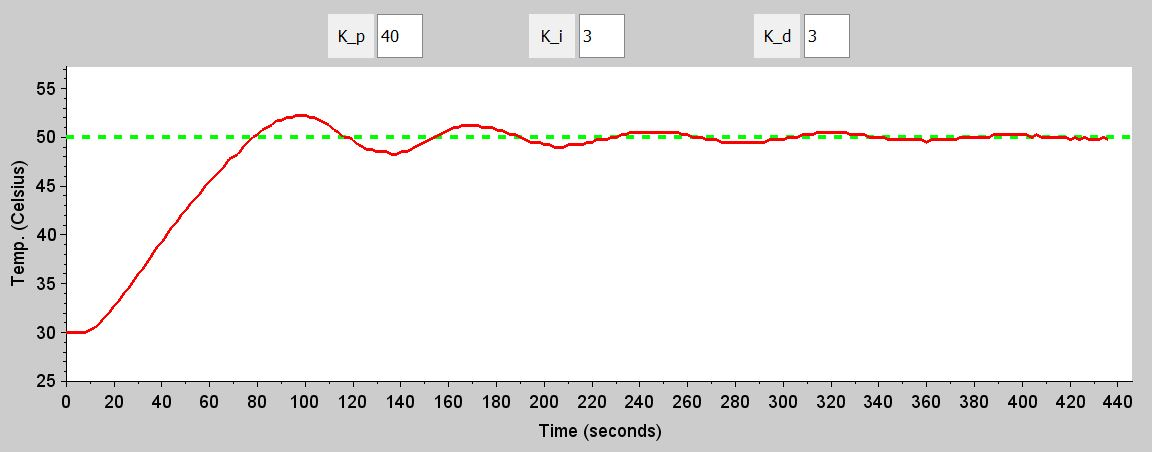Let’s say that the output has converged when the temperature curve stays immediately adjacent to (or directly on top of) the green setpoint line. With the previous configuration (KP = 40/KI = 3/KD = 0), the output never quite converged after about 430 seconds. With KD = 1, the results are about the same. With KD = 2, we have convergence at maybe 400 seconds, and the KD = 3 system seems to converge a little more quickly.

### Conclusion

At this point I am happy with the KP = 40/KI = 3/KD = 3 configuration. In the next article we will look at a more formal technique for tuning the gain values.

Next Article in Series: Embedded PID Temperature Control, Part 6: Ziegler–Nichols Tuning• B
bgwiz March 03, 2016

Odd, I would have thought the KP = 40/KI = 2/KD = 0 configuration chart looked far more desirable at settling out faster than your KP = 40/KI = 3/KD = 3 configuration did, no?

Like.
•RK37 March 05, 2016
The KP = 40/KI = 2/KD = 0 run settles faster, yes, but there is significant steady-state error. The measurement temperature stabilizes at about 1 deg C below the setpoint, because there is not enough integral gain. The KP = 40/KI = 3/KD = 3 run settles more slowly, but the measured temp is centered around the setpoint.
Like.
•SalceyForest March 05, 2016

I’ve rarely used a PID controller for temperature, more usually pressure or motor speed. I have never once used the D term, mostly because I didn’t really understand how to set it properly, but also because noise of various sorts rendered it impossible to use. Any system incorporating inverter motor speed controls anywhere is a total nightmare for delicate transducer signals for instance, but there are also physical artefacts such as air or hydraulic solenoid ‘banging’ which can crop up. Just be careful out there is all.

Like.